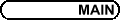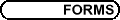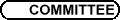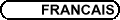Number Theory / Théorie des nombres
Org: Chantal David (Concordia) and/et Andrew Granville (Montreal)

MARK BAUER, University of Calgary, Dept. of Math. & Stats., 2500 University Drive NW, Calgary, AB T2N 1N4
Construction of Hyperelliptic Function Fields of High Three-Rank

In this talk, we will discuss several explicit constructions of hyperelliptic function fields whose Jacobian have high 3-rank. Some of the methods are analogues of techniques for generating quadratic number fields of high 3-rank, while others are unique to the function field setting. In particular, a method for increasing the 3-rank by extending the field of constants will be emphasized, which may also be used to increase the l-rank.

ALINA C. COJOCARU, Princeton University, Dept. of Mathematics, Fine Hall, Washington Road, Princeton, NJ 08544, USA
Exceptional primes for elliptic curves

Let E be an elliptic curve defined over the field of rationals, and for a prime l, let r(l, E) be the l-adic Galois representation associated to E. If E is without Complex Multiplication, an important result of Serre dating from 1972 asserts that r(l,E) is surjective for large enough l. But how large is large enough? This is still an open question, raised by Serre, and investigated, over the years, by Serre, Mazur, Masser and Wüstholz, Kraus, Duke, A. C. Cojocaru, and others.

We say that l is an exceptional prime for E if r(l, E) is not surjective. In this talk, we will show that any one-parameter family of elliptic curves over the rationals has very few exceptional primes. This is joint work with Chris Hall (Univ. Texas at Austin).

JEAN-MARIE DE KONINCK, Université Laval, Dept. de mathématiques et de statistique, Quebec G1K 7P4
Esthetic numbers and game theory

Paul and Mary decide to toss a coin 15 times, for money. If HEADS comes up, Paul gives Mary one dollar. If TAILS comes up, Mary gives Paul one dollar. Before they start playing, Paul announces that he only has \$2 and therefore that he cannot afford to lose any more than \$2, meaning that if he ever reaches a loss of \$3, he will have to quit the game. Mary is more audacious, revealing that she is willing to lose as much as \$4. What's the probability that, under these conditions, they can complete the game? As we shall see, the problem boils down to counting q-esthetic numbers, namely those positive integers whose consecutive digits in base q differ by exactly one.

KARL DILCHER, Dalhousie University, Halifax, Nova Scotia
On finite pattern-free sets of integers

For n Î N let the set A Í {1,...,n} have the property that it does not contain any solution x,y,z to the equation s:ax+by=cz, where a,b,c ¹ a+b are fixed positive integers. Such a set A is called s-free. We investigate maximal s-free sets and determine the upper maximal density bound

 D (s) : = limsup æè 1 n max ìí î |A|:A Í {1,...,n} s-free üý þ öø
for a=1 £ b, and c large. As a byproduct we obtain the upper asymptotic density bound for infinite s-free sets A Ì N defined by
 d (s) : = sup ìí î limsup æè 1 n |AÇ{1,...,n}| öø : A Ì N s-free üý þ ,
for all coprime a, b, and c large, which may be considered the limiting case of finite s-free sets.

Joint work with Lutz G. Lucht, Technische Universität Clausthal, Germany.

JACK FEARNLEY, Université de Montréal
The Fundamental Critical Points of Modular Elliptic Curves

The number of fundamental critical points of the modular form associated with an elliptic curve is an upper bound for the analytic rank of the curve. In their paper "Arithmetic of Weil Curves" Mazur and Swinnerton-Dyer show that this bound is sharp for all but 16 curves of conductor less than 424.

The talk will cover aspects of the computation of the fundamental critical points for all curves of conductor less than 4000. In this range the bound is sharp for approximately eighty per cent of the curves.

HARALD HELFGOTT, Université de Montréal, CP 6128, Succ. Centre-Ville, Montreal, QC H3C 3J7
Power-free values of polynomials with prime arguments

Let f Î Z [x] be a polynomial of degree r ³ 3 without roots of multiplicity r or (r-1). Assume that f(x) \not º 0 mod pr-1 has a solution in (Z/pr-1)* for every p. Erdös conjectured that f(p) is then free of (r-1)-th powers for infinitely many primes p. I prove as much for every f a root of which generates its splitting field, and for some other f as well.

Applications of explicit zero-free regions.

I will discuss number theoretic applications of explicit zero-free regions of Dirichlet L-functions.

WENTANG KUO, University of Waterloo, 200 University Ave. West, Waterloo, ON
A Remark on the Sato-Tate Conjecture

The original Sato-Tate Conjecture concerns the angle distribution of the eigenvalues arisen from non-CM elliptic curves. In this talk, we formulate a modular analogue of the Sato-Tate Conjecture and prove that the angles arisen from non-CM holomorphic Hecke eigenforms with non-trivial central characters are not distributed with respected to the Sate-Tate measure for non-CM elliptic curves. Furthermore, under a reasonable conjecture, we prove that the expected distribution is uniform.

YOUNESS LAMZOURI, Université de Montréal, Département de mathématiques et de statistique, CP 6128, Succ. Centre-Ville, Montréal, QC H3C 3J7
Smooth numbers and delayed integral equations

Let P be a set of primes and define S(x,P) as the set of integers less than x having all their prime factors in P. Let Y(x,P) = |S(x,P)| and p(x,P) be the number of primes less than x for which p-1 is in S(x,P). A difficult but interesting question is to have estimates for the function p(x,P).

A well-know conjecture is that [(p(x,P))/(p(x))] ~ [(Y(x,P))/(x)], under some conditions on the set P. Moreover Granville and Soundararajan proved in 2002 that estimates for Y(x,P) depend on the solutions of the delayed integral equation us(u) = ò0u s(u-t) c(t)  dt. In this talk we prove a general version of the above conjecture assuming the Elliot-Halberstam conjecture, study solutions of the integral equation to obtain estimates for p(x,P), and apply our results to get asymptotics for the set of integers n less than x for which the k-th iterate of the Euler function fk(n) is smooth.

JUNG-JO LEE, Queen's University, Kingston, ON K7L 3N6
Ranks of elliptic curves

There have been many conjectures and results on the ranks of elliptic curves so far. We will see some of those conjectures and the related results especially over the family of quadratic twists of an elliptic curve.

CLAUDE LEVESQUE, Université Laval
Systèmes fondamentaux d'unités de composés de deux corps cubiques

Nous exhibons une famille de composés F de deux corps cubiques pour laquelle nous pouvons exhiber un système fondamental d'unités à partir de la connaissance des unités des sous-corps cubiques de F.

YU-RU LIU, University of Waterloo
A prime analogue of Erdös-Pomerance's conjecture for elliptic curves

Let E/Q be an elliptic curve of rank ³ 1 and b Î E(Q) a rational point of infinite order. For a prime p of good reduction, let gb(p) be the order of the cyclic group generated by the reduction [`(b)] of b modulo p. We denote by w(gb(p) ) the number of distinct prime divisors of gb(p). Assuming the GRH, we show that the normal order of w(gb(p) ) is loglogp. We also prove conditionally that there exists a normal distribution for the quantity

 w æè gb(p) öø - loglogp

 Ö loglogp
.
The later result can be viewed as an elliptic analogue of a conjecture of Erdös and Pomerance about the distribution of w(fa(n) ), where a is a natural number > 1 and fa(n) the order of a modulo n.

SAID MANJRA, University of Ottawa
On the E-functions

The E-functions were introduced around 1929 by C. L. Siegel who studied the irrationality and transcendence of values at the algebraic points. The minimal differential operator annihilating an E-function is called E-operator. In this lecture, we give an arithmetic characterization of such operator based on a recent results of Yves André.

DAVID MCKINNON, University of Waterloo
Rational Approximation on Algebraic Varieties

If two rational points in affine space have small height, then they must lie on a rational line with small height-that is, the line connecting the two points has coefficients with small height. Thus, if one wishes to approximate a rational point P with other rational points, the best way to do it is to use rational points which lie on lines of small height through P.

What happens if one constrains the approximating points to satisfy a set of polynomial relations? This question is still open, but the evidence so far points to a somewhat surprising answer.

ROGER PATTERSON, University of Calgary, 2500 University Drive NW, AB, T2N 1N4
Sequences of rational torsion on Jacobians

We will construct sequences of hyperelliptic curves of genus g whose Jacobians possess torsion divisors of order Q(g2). These families generalize the earlier examples of Flynn and Leprévost.

GUILLAUME RICOTTA, Université de Montréal, Bureau 6213, Département de mathématiques et de statistique, CP 6128, Succ.  Centre-Ville, Montréal, QC H3C 3J7
On non-trivial real zeros of L-functions

In 2002, J. B. Conrey and K. Soundararajan have shown that there are infinitely many Dirichlet L-functions which do not vanish on the critical segment. Two crucial remarks about their result. On one hand, the analytic technique used (mollification method) and their zeros counting method compel them to get strong asymptotic formula for the mollified second moment of their family of Dirichlet L-functions at a distance of the inverse of the logarithm of the analytic conductor of their family. On the other hand, all their work is justified by the random matrix model of their family: its symmetry type is the symplectic one which entails a repulsion of the first zero a little away from the critical segment. Following their work, a similar analytic study of a family of Rankin-Selberg L-functions which has the same symmetry type has been undertaken, but this time a deep and tough new problem occurs: namely the resolution of a shifted convolution problem on average. The two main purposes of this short talk will be to describe and legitimize the mollification method which is not only a cunning craftiness and to give some indications on shifted convolution problems.

DAMIEN ROY, University of Ottawa
Criteria of algebraic independence

We review some of the present criteria of algebraic independence and discuss avenues for further research.

MICHAEL RUBINSTEIN, University of Waterloo, 2000 University Ave. W, Waterloo, ON N2L 3G1
Announcing the L-function Calculator

I'll describe the L-function calculator, and present some of the algorithms that lie behind it.

RENATE SCHEIDLER, Dept. of Mathematics & Statistics, University of Calgary, 2500 University Drive NW, Calgary, AB T2N 1N4
Class Number Approximation in Cubic Function Fields

A common way of computing the order h of the Jacobian Jac(Fq) of an algebraic function field K over a finite field Fq is to determine an interval ] E-L,E+L[ that is known to contain h and then search this interval using a baby step giant step technique or Pollard's kangaroo method. In the case where K/Fq(x) is a cubic extension, we use approximations via truncated Euler products to explicitly compute suitable values of E and L, thereby giving an algorithm for finding h that has complexity O(q(2g-1)/5) where g is the genus of K/Fq.

This is joint work with A. Stein of the University of Wyoming.

CAM STEWART, University of Waterloo
On prime factors of n!+1

By Wilson's Theorem if p is a prime then p divides (p-1)!+1. In 1856 Liouville proved that (p-1)!+1 is not a power of p if p exceeds 5. In 1976 with Erdos we investigated the greatest and least prime factors of integers of the form n!+1. We shall discuss these topics and related recent results of Luca and Shparlinski and of the speaker.

ZHI-HONG SUN, School of Mathematics and Statistics, Carleton University, Ottawa, ON K1S 5B6 (from Huaiyin Teachers College in China)
Quartic residues and binary quadratic forms

Let p be a prime of the form 4k+1, m Î Z and p\nmid m. In this talk we give a general criterion for m to be a quartic residue of p in terms of appropriate binary quadratic forms. Let d > 1 be a squarefree integer such that ([(d)/(p)])=1, where ([(d)/(p)]) is the Legendre symbol, and let ed be the fundamental unit in the quadratic field Q (Öd). Since 1942 many mathematicians tried to characterize those primes p so that ed is a quadratic or quartic residue of p. Now we completely solve these open problems by determining the value of (u+vÖd)(p-([(-1)/(p)]))/2 mod p), where p is an odd prime, u,v,d Î Z, v ¹ 0, gcd(u,v)=1 and ([(-d)/(p)])=1. As an application we obtain a general criterion for p | u(p-([(-1)/(p)]))/4 (a,b), where {un(a,b)} is the Lucas sequence defined by u0=0, u1=1 and un+1 = bun-aun-1 (n ³ 1). In the talk we also present the exact value of the number of incongruent residues of x4+bx modulo an odd prime.

ALAIN TOGBE, Purdue University North Central, 1401 South U.S. Highway 421, Westville, IN 46391
Complete Solutions of a Family of Cubic Thue Equations

In this talk, we will consider a family of Thue equations associated with a family of number fields of degree 3. First we will give details about the number field and then we will show how we use Baker's method to completely solve this family of Thue equations.

GARY WALSH, University of Ottawa, 585 King Edward Avenue, Ottawa, Ontario K1N 6N5
Quantitative results for Diophantine m-tuples of polynomials

A D(n)-m-tuple is defined to be a set of integers {a1, ...,am} with the property that for all 1 £ i < j £ m, aiaj+n is a perfect square.

In recent years there have been many new results on the number of elements in such sets, most notably by A. Dujella, who essentially settled on old problem, dating back to Diophantus, by proving that there are no D(1)-6-tuples, and only finitely D(1)-5-tuples.

More recently, Dujella, Fuchs and Tichy proved upper bounds in the case that the ai are polynomials of equal degree, and n is a linear polynomial.

We will present quantitative improvements to these results, along with specific examples, which together imply that the new upper bounds are sharp. This is joint work with Andrej Dujella and Clemens Fuchs.

HUGH C. WILLIAMS, Mathematics and Statistics, University of Calgary, 2500 University Drive NW, Calgary, AB T2N 1N4
Computing the Regulator of a Real Quadratic Field

One of the most important invariants of a real quadratic number field is its regulator R. The problem of computing R is very old and very difficult, particularly when the field discriminant D becomes large. (Clearly, the actual value of the regulator can never be computed because it is a transcendental number; we are content with an approximate value that is within 1 of the actual value.) The best current method for computing R is Buchmann's subexponential method. Unfortunately, the correctness for the value of R produced by this technique is dependent on the truth of a generalized Riemann hypothesis. The best unconditional algorithm (the value of R is unconditional, not the running time) for computing the regulator of a real quadratic field is Lenstra's O(D1/5+e) Las Vegas Algorithm. In this talk we describe a technique for rigorously verifying the regulator produced by the subexponential algorithm. This technique is of complexity O(D1/6+e). Furthermore, these methods can be extended to the problem of determining rigorously for real quadratic fields of large discriminant whether a given ideal is principal. This is of great importance in solving certain Diophantine equations.

Joint work with Robbert de Haan and Mike Jacobson.

GUANGWU XU, University of Toronto, Toronto, Ontario M5S 3G3